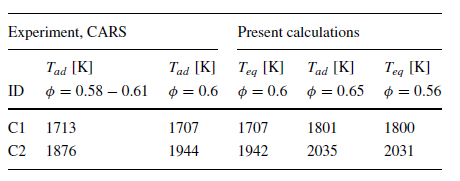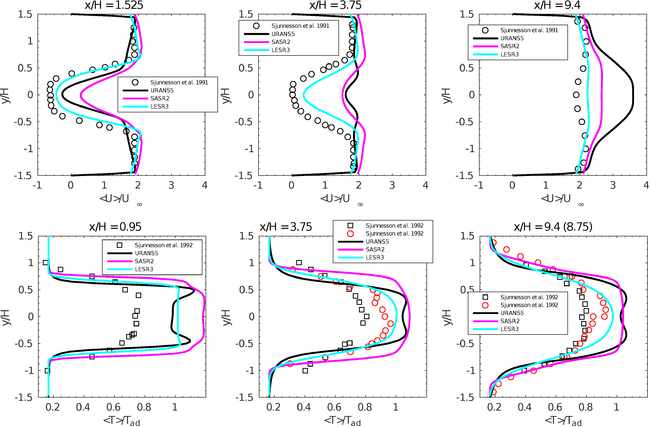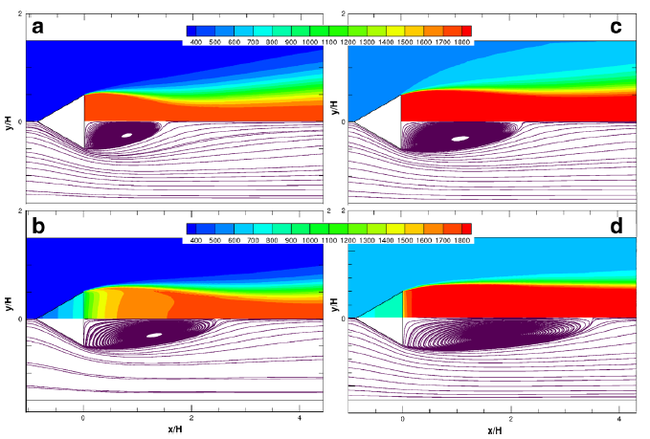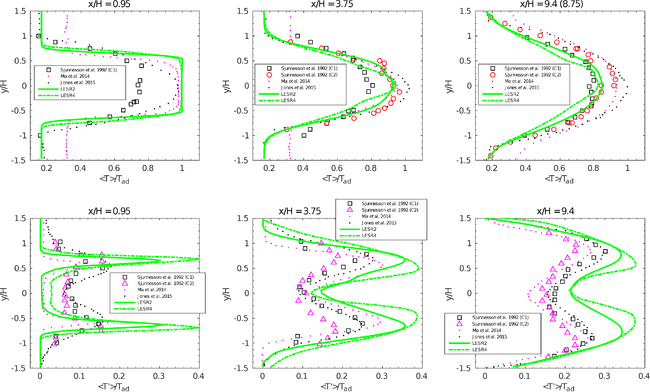# Turbulent separated inert and reactive flows over a triangular bluff body

## Key Fluid Physics

The Reynolds numbers based on the side of the bluff-body and bulk velocity are estimated as Re=28,000 – 47,000, and the flow can be considered to be in the sub-critical regime for the inert simulations. The combustion is characterized by the lean, premixed propane-air mixture of equivalence ratio φ = 0.58 – 0.65 (“thin reaction zone” regime). The key features of the flow mechanics are the laminar boundary layer, separated shear layer, wake and the flow instabilities that provide complex, nonlinear interactions between them. The wake is dominated by two types of instabilities: the convective instabilities or asymmetric vortex shedding the (Bénard/von Kármán instability) and Kelvin–Helmholtz instability (sometimes called absolute) of the separated shear layer. For the reactive cases, the flame introduces additional phenomena trough effects of exothermicity and flow dilatation on the flow field, which leads to the large differences between the non-reacting and the reacting wakes.

## Application Uncertainties

A word of caution should be given here concerning the estimation of the adiabatic flame temperature since two sets of experiments were performed: the CARS measurements  and the gas analysis . Sjunnesson et al.  provided the adiabatic temperatures for the CARS measurements (φ = 0.58 – 0.61) as Tad = 1713 K and Tad = 1876 K for the cases C1 and C2, respectively. However, all present numerical results were calculated for the conditions with φ = 0.65 relevant to the gas measurements . The estimated adiabatic temperatures for the cases C1, C2 and φ = 0.65 were Tad = 1800 K and Tad = 2035 K, respectively, which were used in the previous sections for all figures to normalize temperature. The calculated adiabatic temperatures were consistent with the temperatures calculated on the basis of the chemical equilibrium assumption (Teq) as well. Table 7 summaries all these findings.Table 7: Adiabatic and chemical equilibrium flame temperatures for C1 and C2 flow conditions

## Discretisation and Grid Resolution

Concerning discretization errors both spatial and temporal discretization errors should be considered. Usually the spatial discretization error effects are larger than the errors arising from the time integration . It can be shown that in the case of fully developed turbulent flows, existing small time and space scales are simply advected by the most energetic eddies . This argument yields an accuracy time-scale similar to the Courant-Friedrichs-Lewy (CFL) criterion. Thus, in all present calculations, the stability condition CFL < 0.75 was employed, which guaranteed that the actual time step was close to the accuracy time step. Several calculations were performed to investigate the spatial discretization error of the present SAS and LES results. It was interesting to see that the non-reactive SAS results obtained on the refined mesh provided some improvement in the accuracy of the mean flow field, both for the first and second order statistics, while at the same time keeping the similarity in the flow dynamics (energy spectra and vortex shedding).On the other hand, the reactive SAS calculations did not show any significant improvement in the predictions for the mean flow field as well as the wake dynamics. The results obtained by the combustion LES on the two grids showed some improvement in the accuracy for the second order statistics, while the first order statistics and wake dynamics were captured approximately with the same level of precision. Finally, satisfactory agreement was obtained between the measured and resolved turbulence kinetic energy by the present SAS and LES, which indicates, at least qualitatively, that the spatial resolution of the used grids was adequate.

## Physical Modelling

From the modeling point of view, the following groups of physical processes related to the complex combustion phenomena have to be modeled: the turbulence, the chemistry, the turbulence-chemistry interaction and the heat and mass transfer.

### Overview of capabilities to predict turbulent combustion by URANS, SAS and LES

Figure 26 displays the spatial profiles of the mean stream-wise velocity and the mean temperature at three different locations in the wake obtained by the different modeling approaches. All models have some kind of interrelation and similarity in the estimation of the turbulence kinetic energy by using a separate transport equation, although the viscous dissipation is treated in a different manner. The most accurate prediction of the recirculation zone length was obtained by the LES-EDC closure, while the SAS and URANS results significantly under-estimated Lr. This observation was made in spite of the fact that both URANS and SAS calculations showed very satisfactory agreement for the inert flow conditions. The discrepancies between URANS, SAS and LES for the temperature and other scalar fields are of similar nature. In general, the present LES-EDC closure provided superior results over the URANS and SAS models. Also, it can be seen clearly that URANS and SAS calculations yielded a sharper spatial distribution of the temperature compared to the parabolic-like shape predicted by LES-EDC. At the location x/H = 0.95, all three models yielded significant over-estimation of the flame temperature, mostly due to the simplified treatment of the heat and mass transfer effects. Further downstream, at the locations x/H = 3.75 and 9.4, the temperature profiles obtained by URANS, SAS and LES have similar qualitative behavior. Nevertheless, URANS and SAS yielded sharper profiles with some over-estimation of the peak flame temperature. This can possibly be explained by the excessive viscous dissipation in the shear layers generated by the URANS and SAS models, which consequently results in predictions of unrealistic time and length scales used in the EDC-PSR formalism to calculate reaction rates.Figure 26: Normalized mean stream-wise velocities and temperatures predicted by different modeling approaches: URANS5, SASR2 and LESR3

### Effects of heat and mass transfer

In the present study several RANS/URANS calculations were performed using the EDC and TFC closures with different values of the turbulent Schmidt number. It was observed that for the flow conditions C1 (T = 300 K), Sct = 0.4 provided superior results compared to its conventional value of Sct = 0.85. This finding was consistent with the data available in the literature [20,21,22], and is valid for both the EDC and TFC closures. Further analysis of the numerical results for the C2 operational conditions (T = 600 K) showed that calculations with Sct = 0.85 yielded slightly better agreement with the experimental data than similar runs with Sct = 0.4 , but this observation is only valid in the far wake (at x/H = 0.8 and further downstream).

Figure 27 presents the effect of heat losses on the development of the velocity field and the prime recirculation zone just downstream of the bluff body. Here, limiting cases with the adiabatic (RANS7 and RANS6) and fully-CHT (RANS3 and RANS5) boundary conditions are compared for both flow conditions C1 and C2, respectively. It can be observed that the length of recirculation zone was significantly increased when the heat transfer effects were taken into account for both operational conditions. Thus, the recirculation zone increased from <Lr,ad >/H = 1.492 to < Lr >/H = 2.5 for the flow condition C1 (<Lr,exp>/H = 3.55), and from <Lr,ad>/H = 2.25 to <Lr>/H = 3.58 for the flow condition C2 (<Lr,exp>/H = 3.16), respectively. Finally, one can see clearly that heat transfer effects are important and need to be taken into account in the vicinity of the recirculation zone.Figure 27: Effects of heat losses on the velocity field depending on operational conditions C1 (a – RANS7, b – RANS3) and C2 (c – RANS6, d – RANS5) for the Volvo test rig

### Effects of the turbulence – chemistry interaction

Recent numerical results by Jones et al.  are used to assess the influence of the turbulence-chemistry interaction models for the reacting LES. Jones et al.  studied the Volvo test-rig case using LES and a sub-grid scale probability density function (LES-PDF, hereafter) approach in conjunction with the stochastic - field solution method to account for the subgrid-scale turbulence–chemistry interactions. Numerical results by Ma et al.  are added in spite of the fact that these results have been obtained for the C2 flow conditions (T = 600 K, φ = 0.58). Ma et al.  developed a reaction-rate closure derived from the scalar dissipation rate (LES-SDR, hereafter), which has been assessed based on a simultaneous a priori DNS analysis and an a posteriori LES analysis. The present LES-EDC results (LESR2) based on the conventional Smagorinsky model were chosen for comparison.

Figure 28 compares profiles of the mean temperature and its fluctuation obtained by these LES calculations with present ones. At location x/H = 0.95, LESR2 provided a more sharpened, cone-like mean temperature profile, which over-predicted the measurements. The fluctuations of the temperature obtained by LESR2 are also more sharp in the regions where mixing of fresh reactants and burnt products and combustion takes place. Outside the recirculation zone, at locations x/H = 3.75 and x/H = 9.4, LESR2 yielded approximately both qualitative and quantitative agreement for the mean temperature. For the temperature fluctuations LESR2 provided qualitatively the same trends as the measurements.

The conventional Smagorinsky and the k-equation SGS models were tested for the reactive LES. It was observed that the LES-EDC with the Smagorinsky model provided slightly better results compared to the k-equation SGS model. However, it is worth noting that the flame dynamics revealed by the Smagorinsky model was completely different with intermittency between pockets of fresh and burnt gases that was probably not observed in the experiment. The effects of the Smagorinsky constant were investigated as well and showed that LES-EDC results with Cs = 0.053 provided slightly more precise results compared to Cs = 0.1.Figure 28: Normalized mean and fluctuating temperatures predicted by the LES-EDC (LESR2 and LESR4), LES-SDR , LES-PDF  and experimental data for the Volvo test rig. Experimental data relevant to the LES-SDR (C2) are added for consistency.

## Recommendations for Future Work

The focus of future work should be on the following aspects:

• Several approaches have been discussed, including the conventional RANS/URANS, the large-eddy simulation and the scale-adaptive simulation. However, it will be interesting to assess the detached-eddy simulation (both inert and reactive) for the present benchmark as well;
• The shear layer instabilities were qualitatively captured (based on the flow visualization) for both inert and combustion cases. However, quantitative information (frequencies) was not examined. Thus, it will be useful to estimate the frequencies of the shear layer instabilities and compare them to those for other bluff-bodies (etc., circular cylinder);
• Finally, one of the important aspects of unsteady combustion physics is the lean blowoff (LBO). The simplicity of the test setup makes it a very attractive platform for further investigation of the different LBO modeling approaches.